# 逻辑回归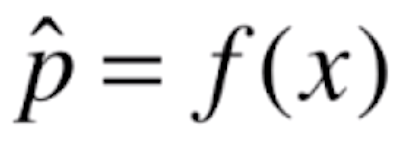，进来一个x，经过f(x)的运算，会得到一个概率值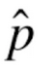。之后我们根据这个概率值来进行分类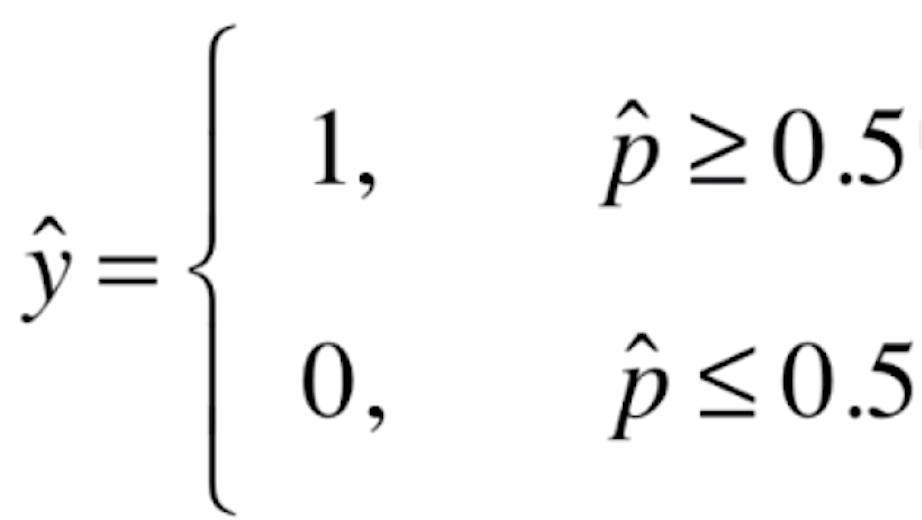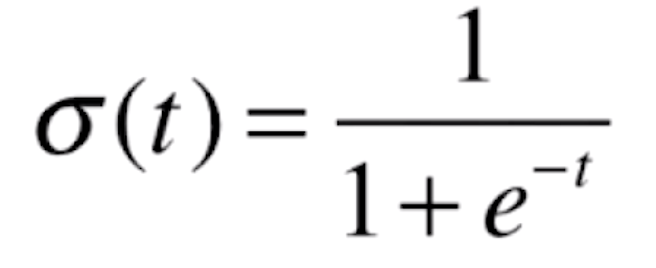```import numpy as np
import matplotlib.pyplot as plt

if __name__ == "__main__":

def sigmoid(t):
return 1 / (1 + np.exp(-t))

x = np.linspace(-10, 10, 500)
y = sigmoid(x)
plt.plot(x, y)
plt.show()```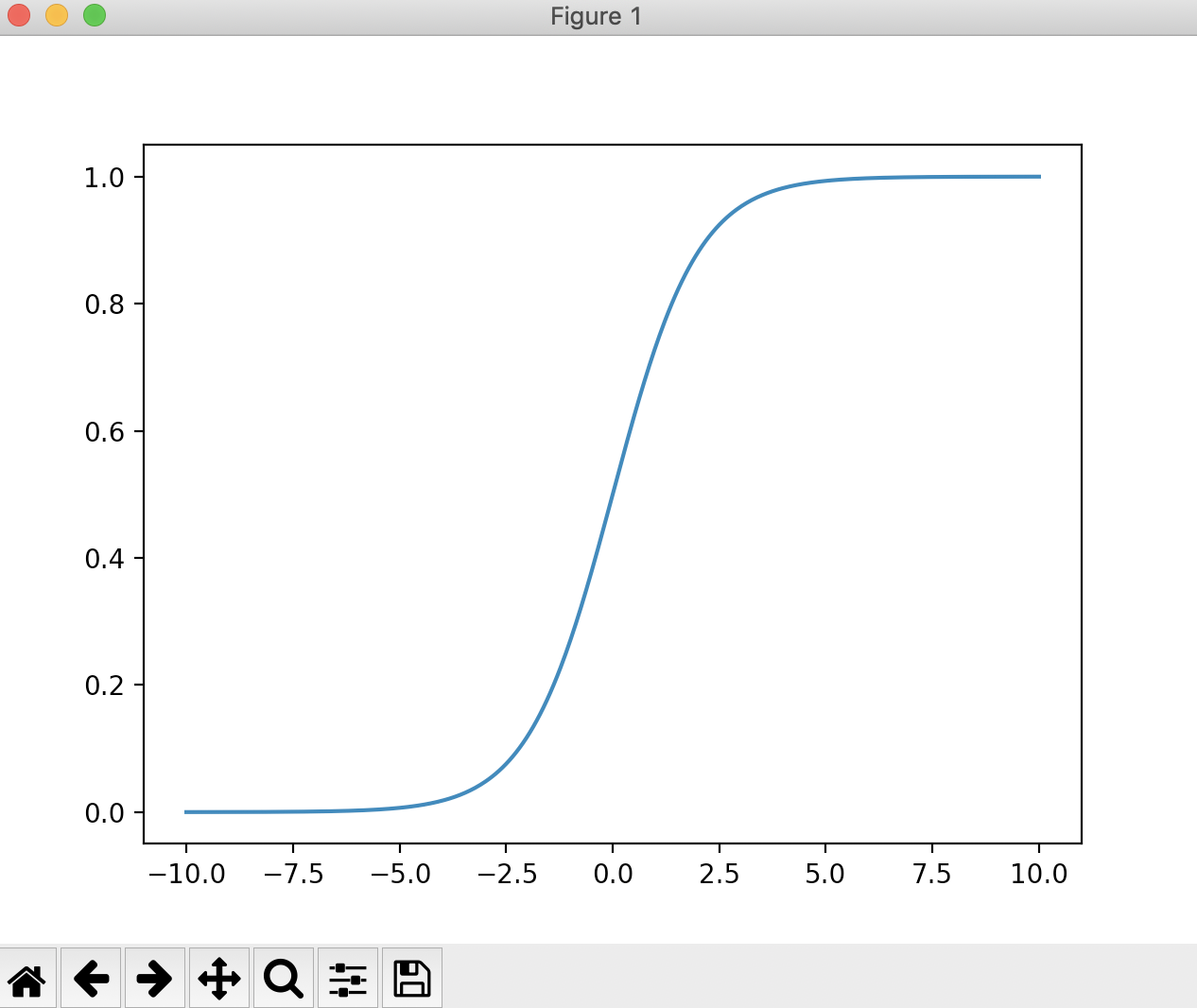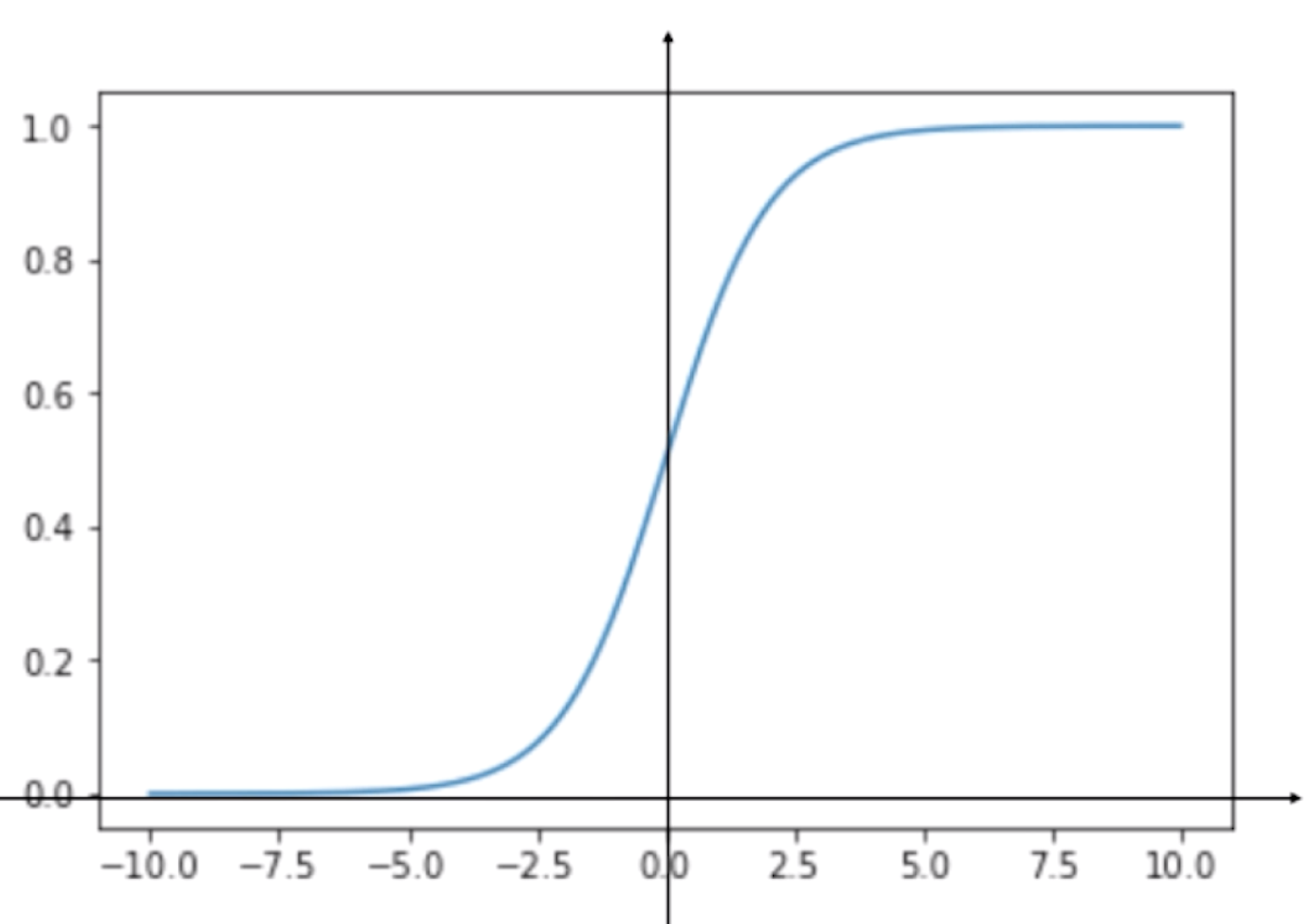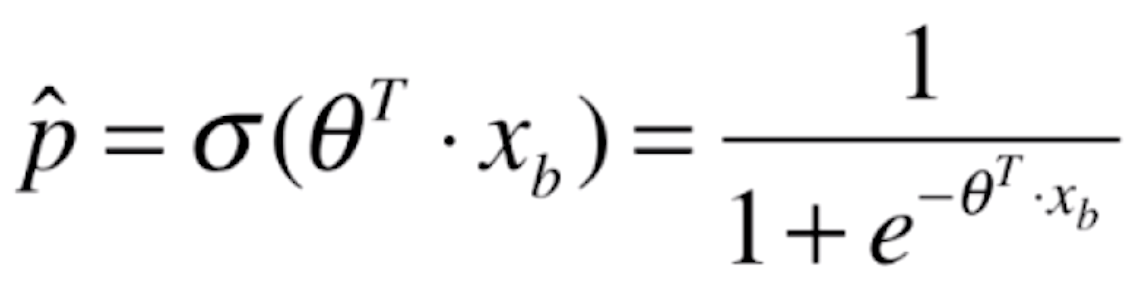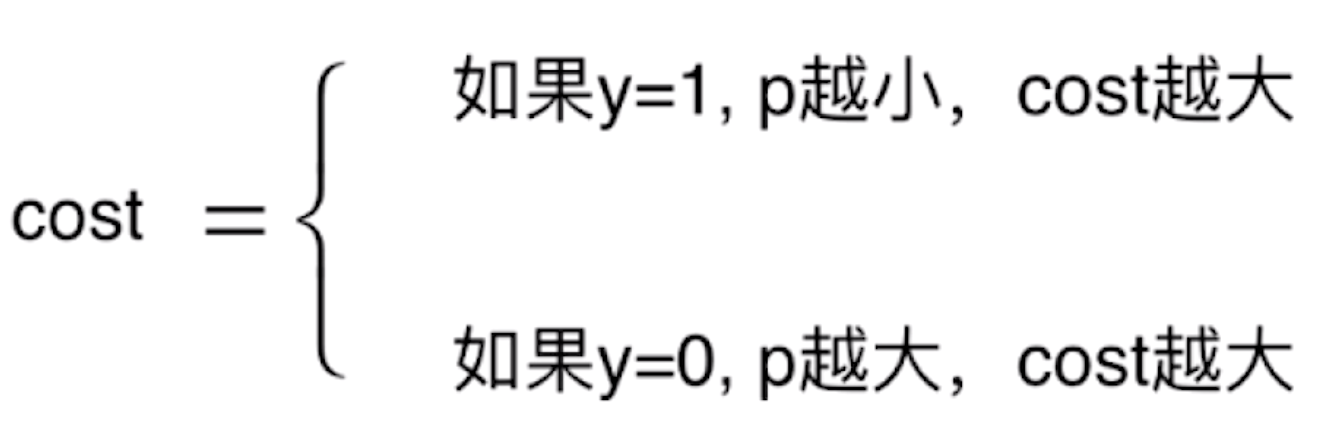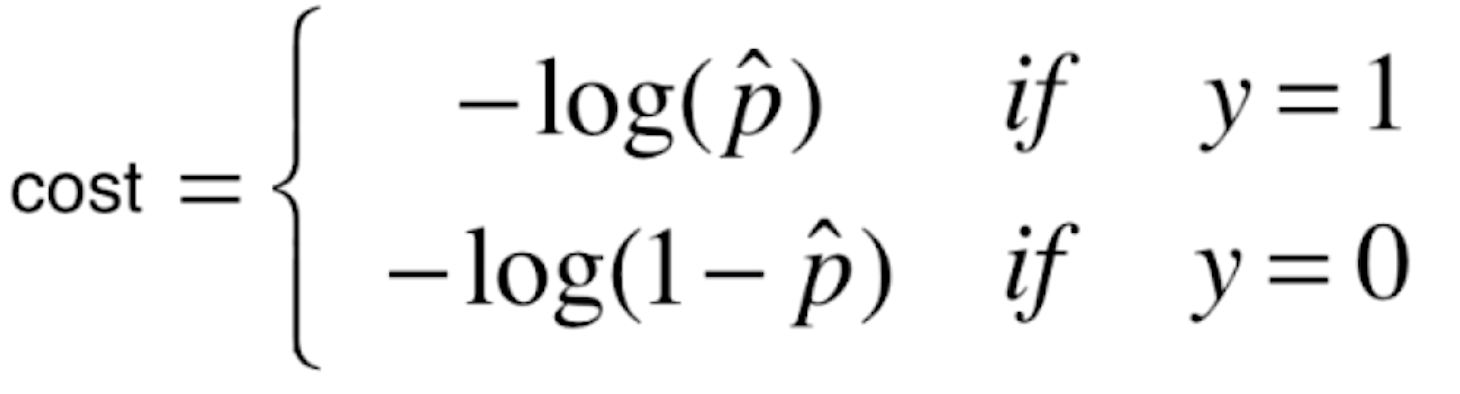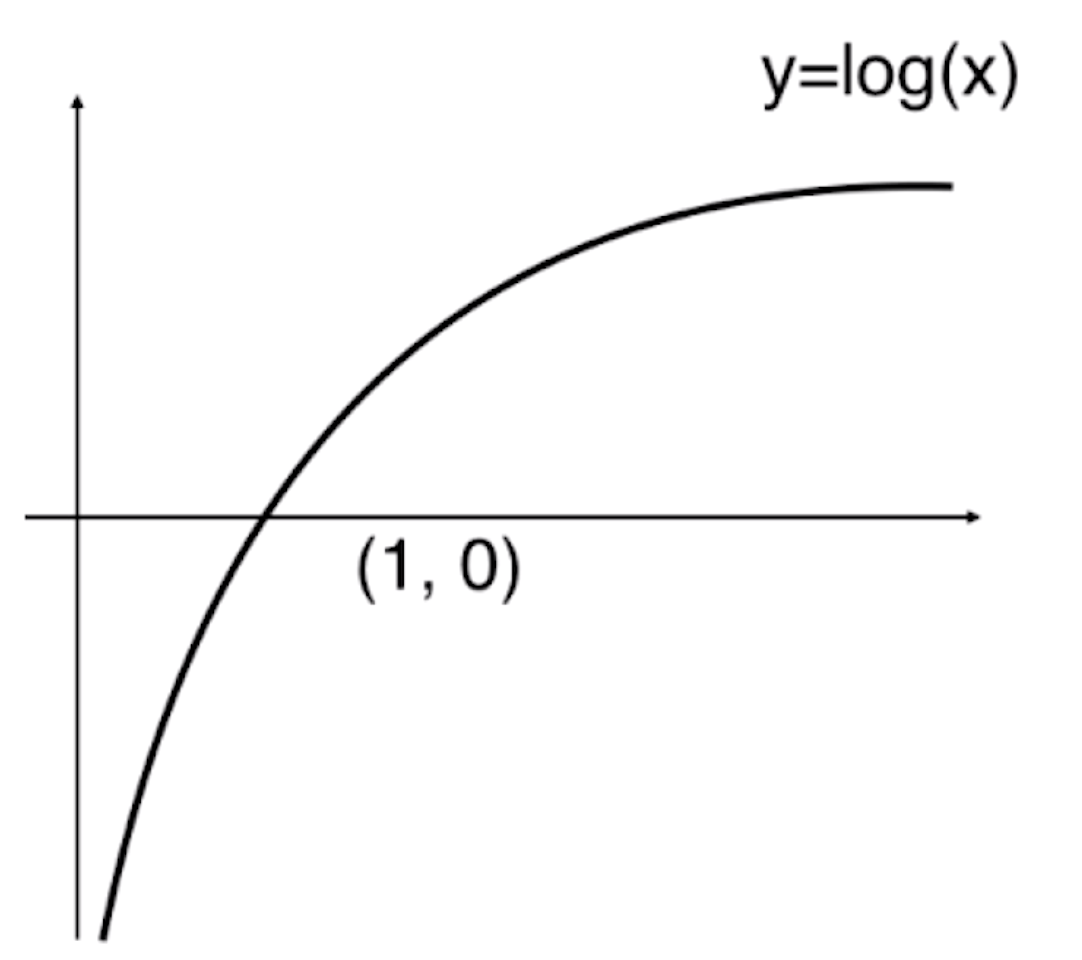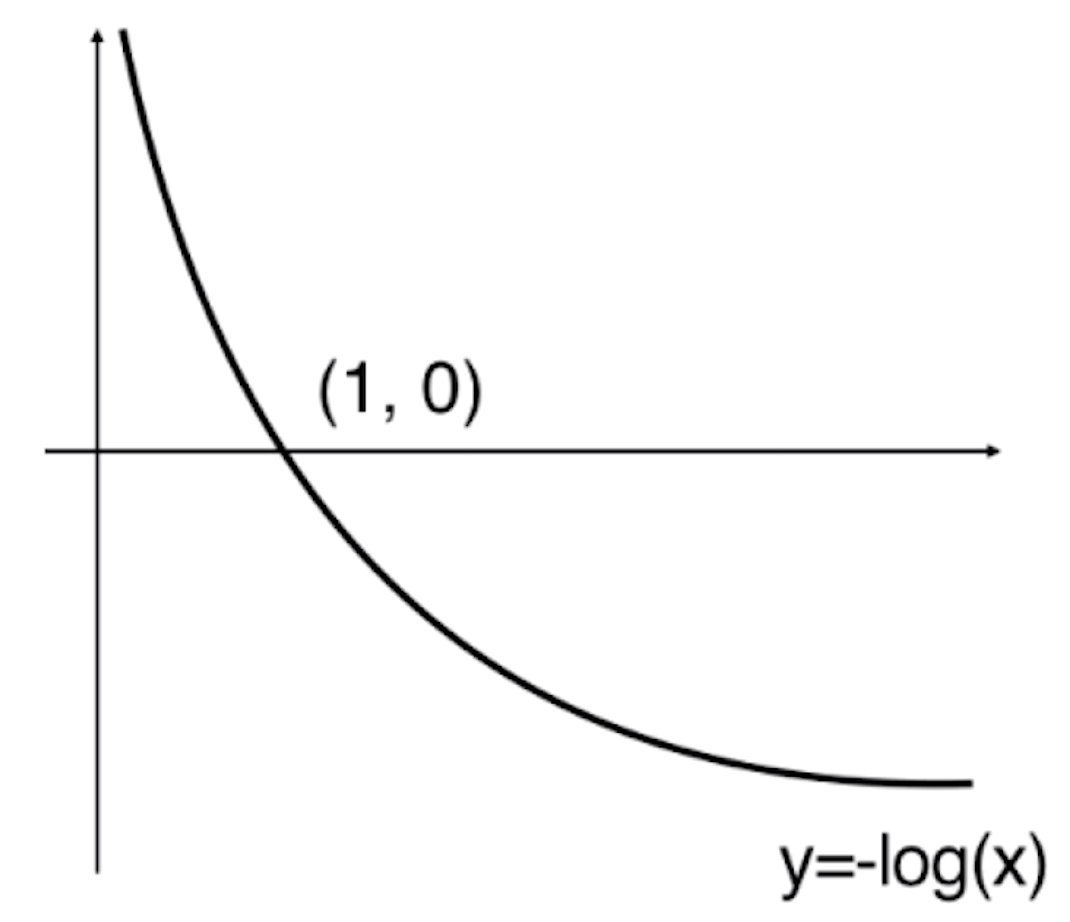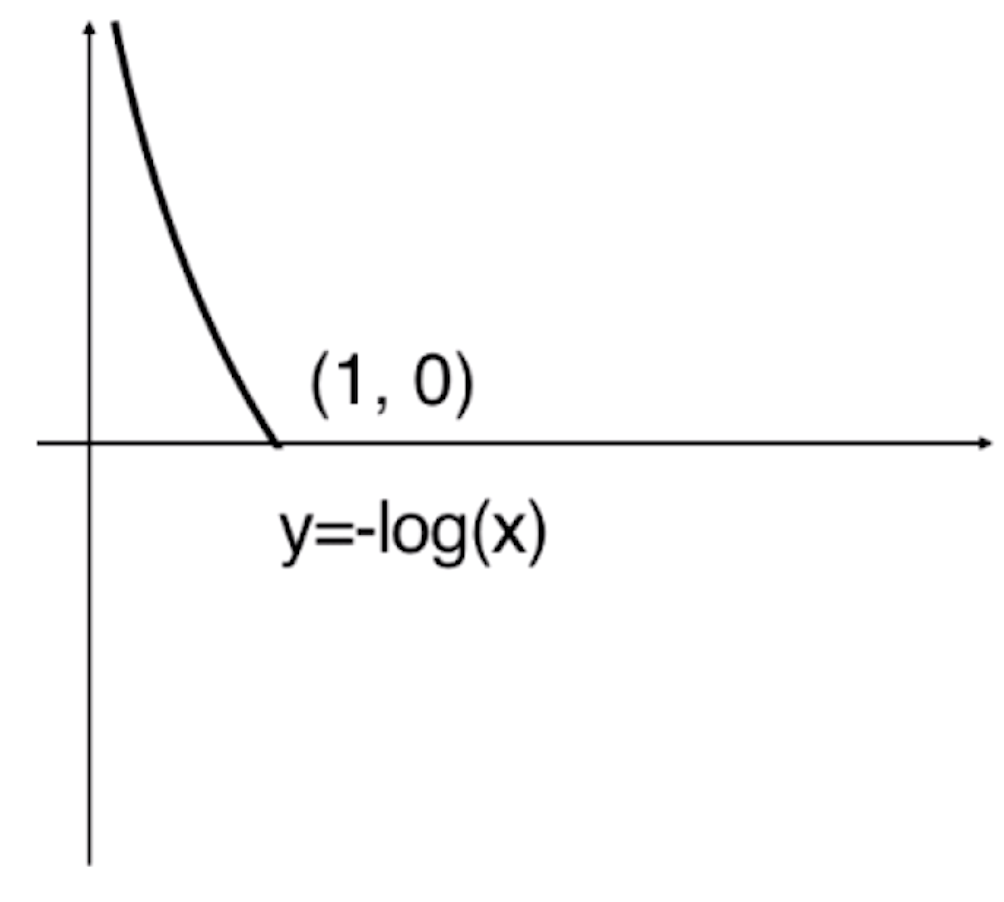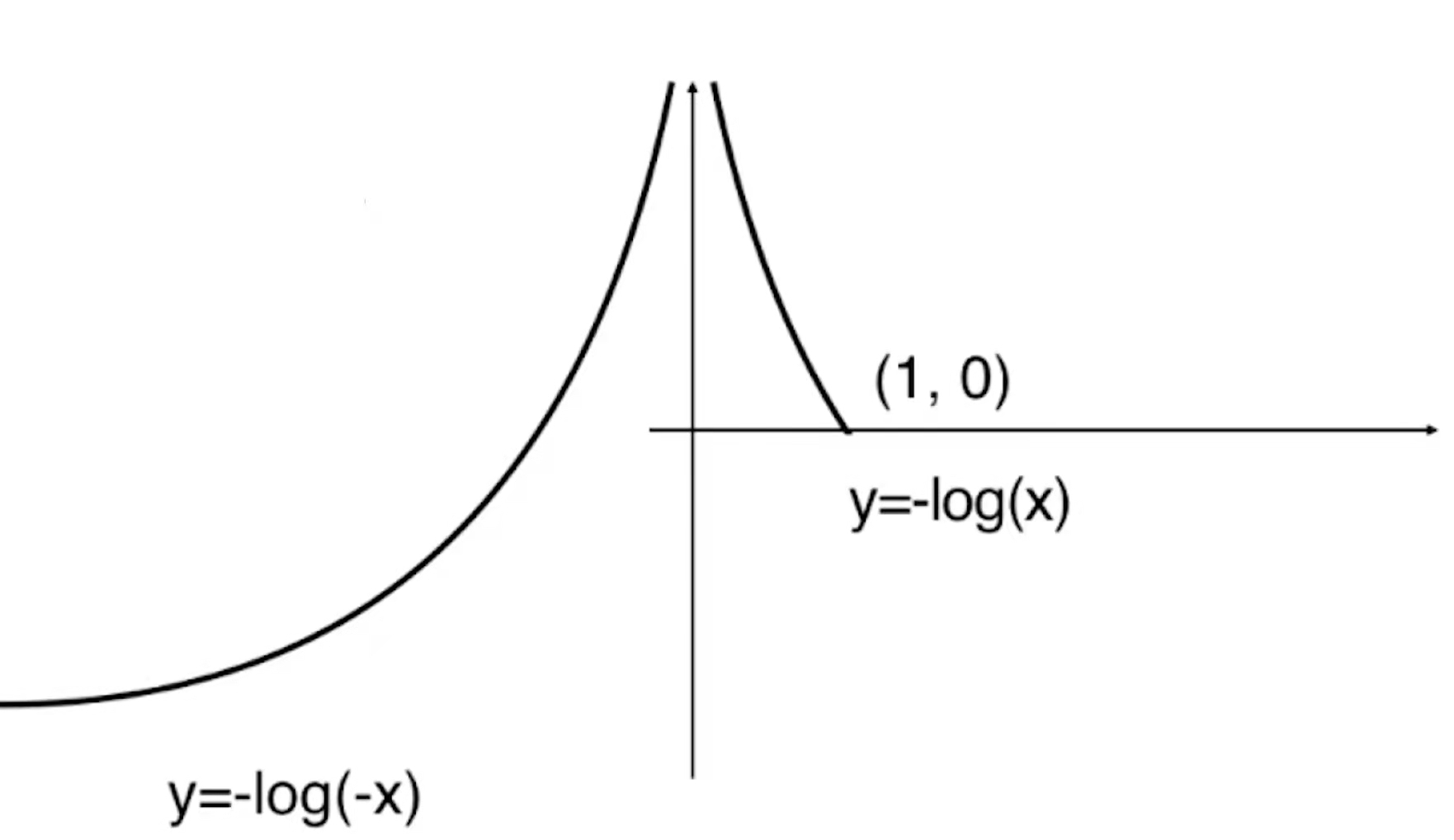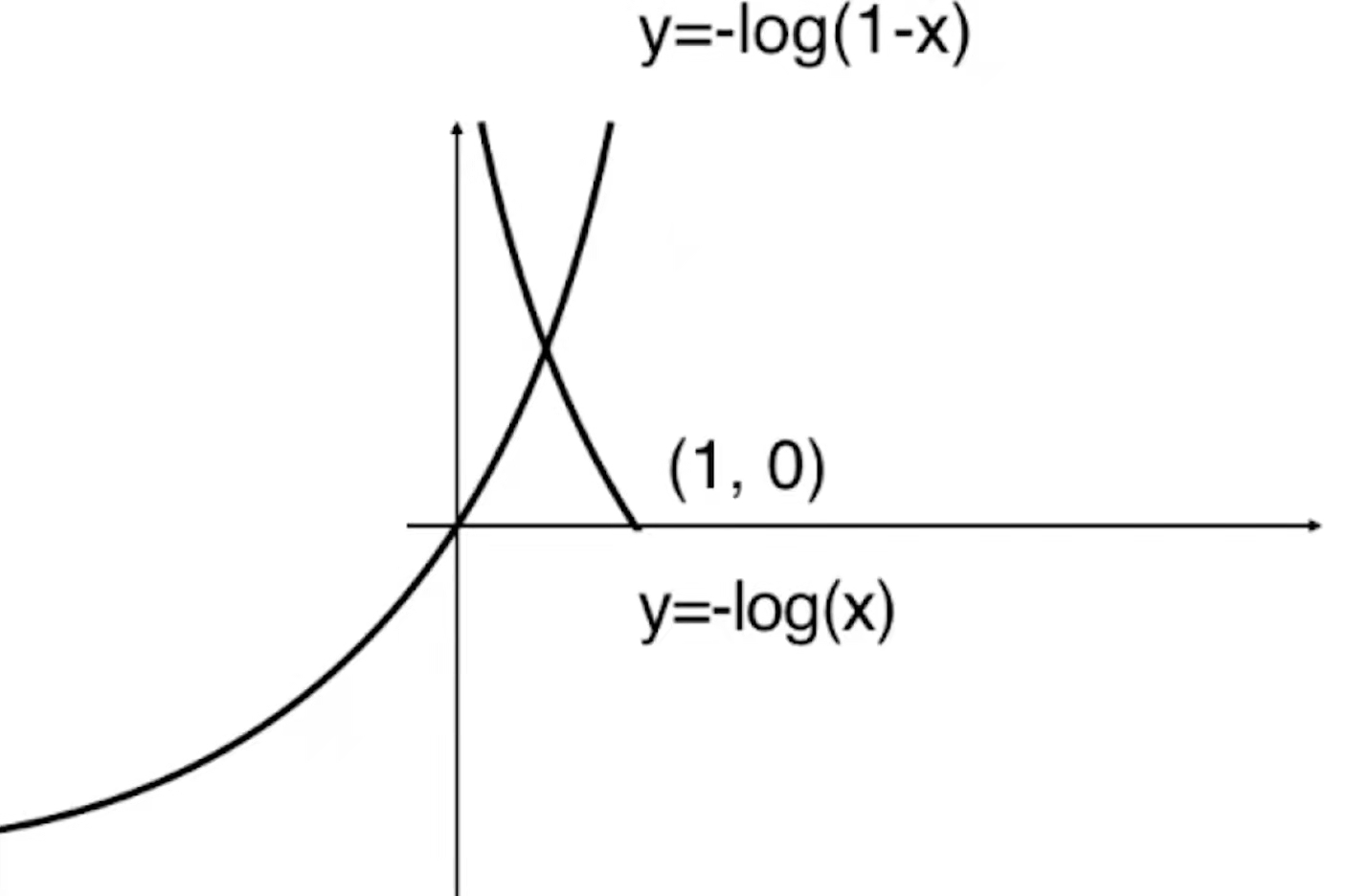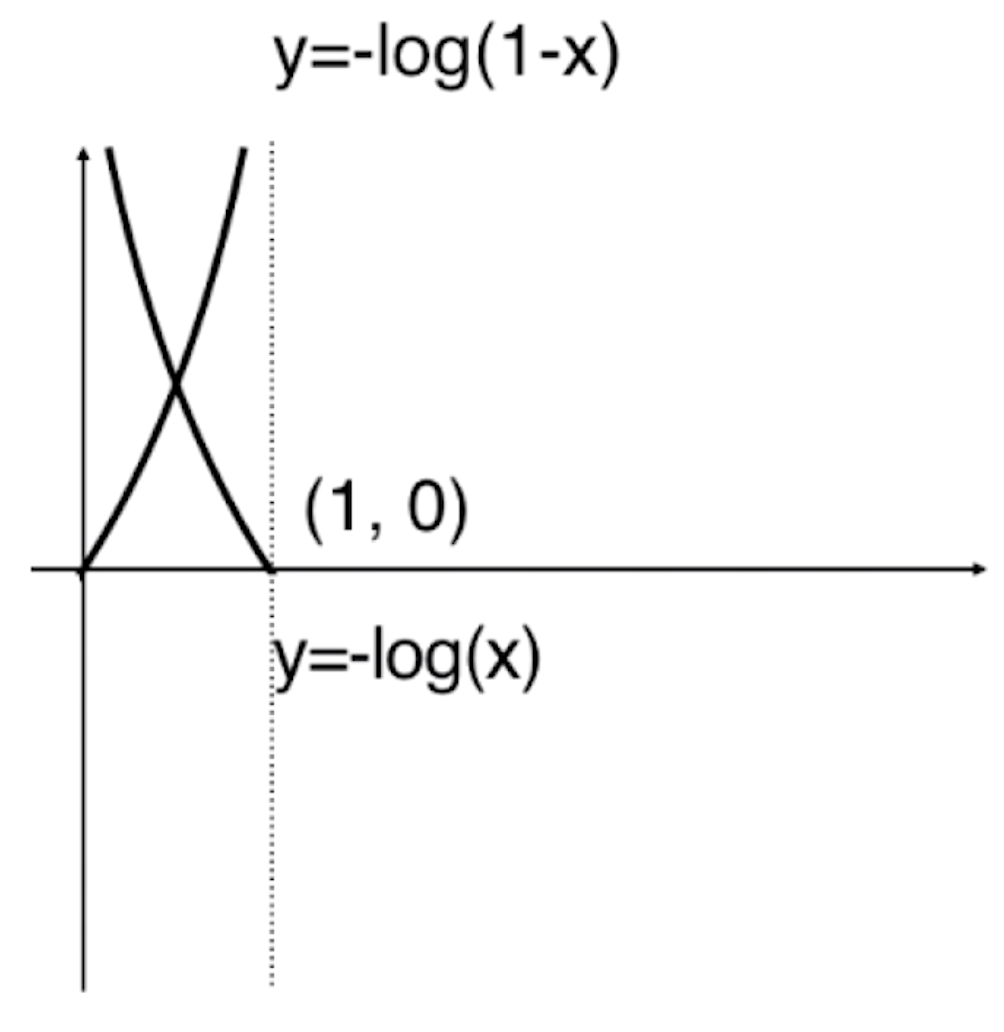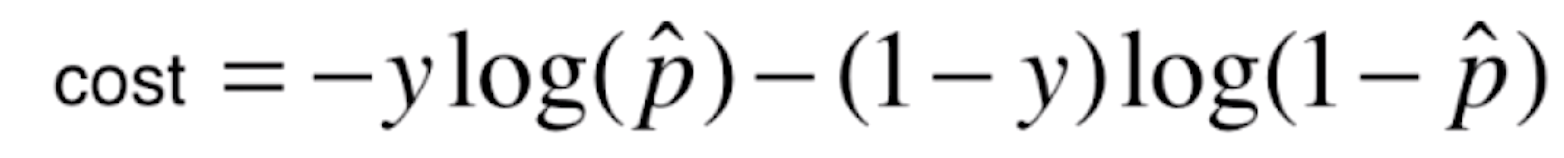，这个函数和上面的分类函数是等价的，原因也很简单，当y=1的时候，该式就等于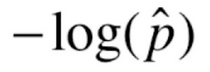，当y=0的时候，该式就等于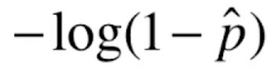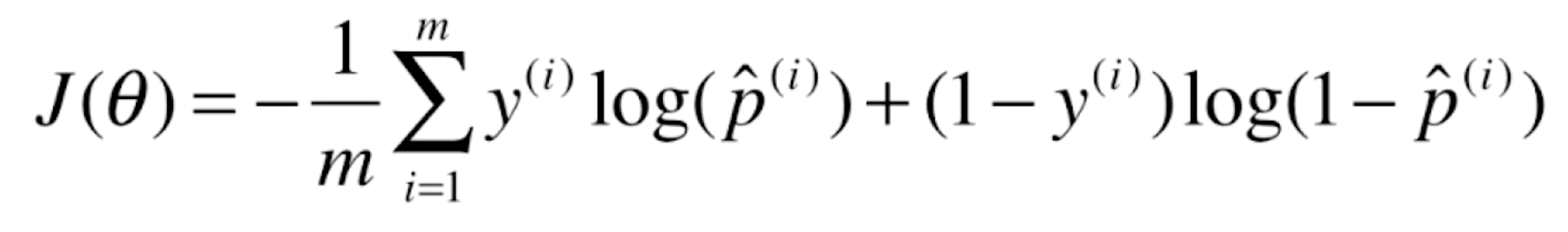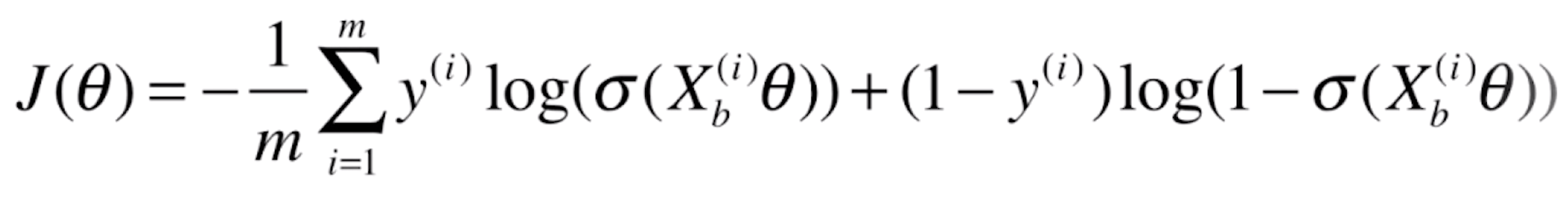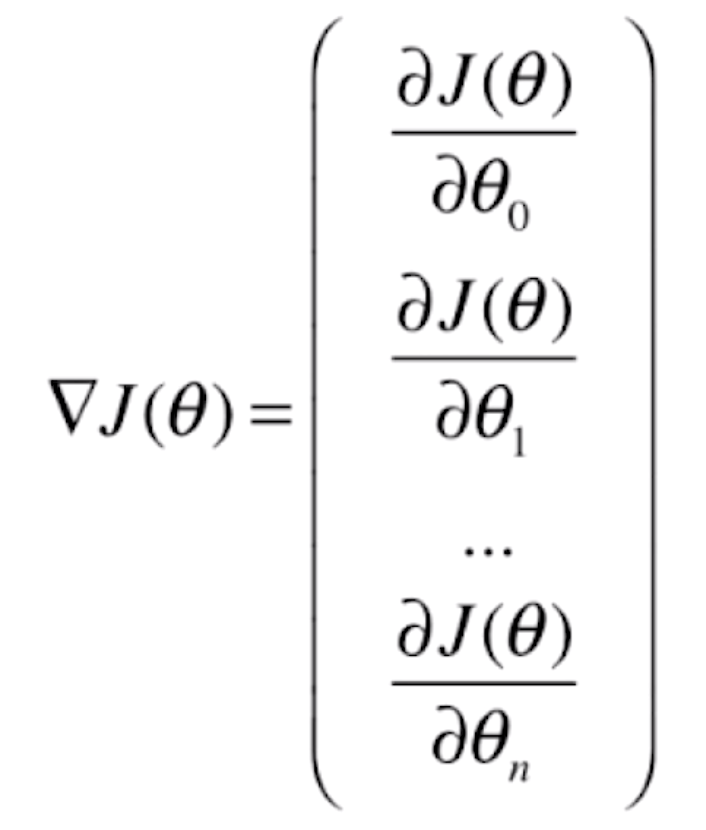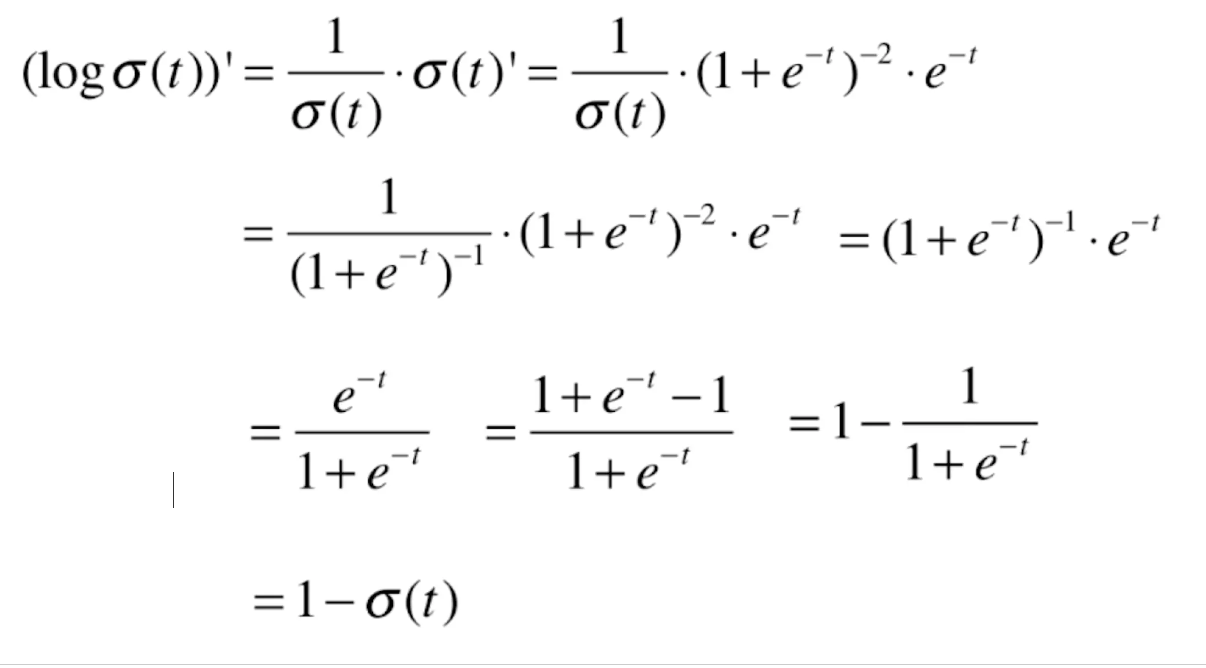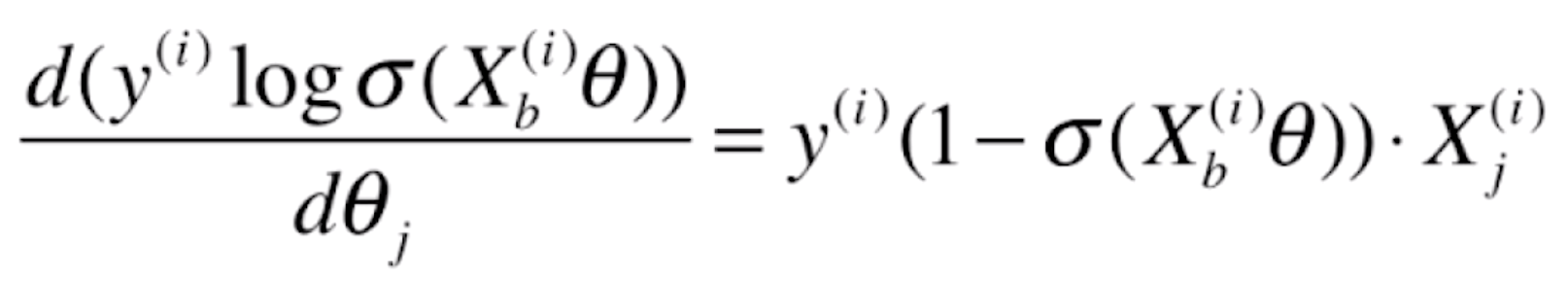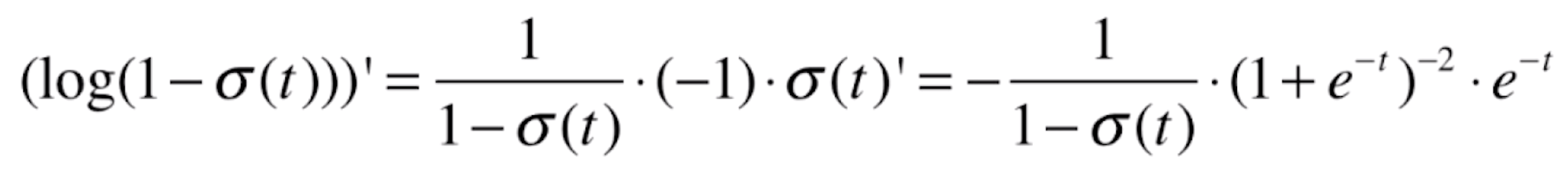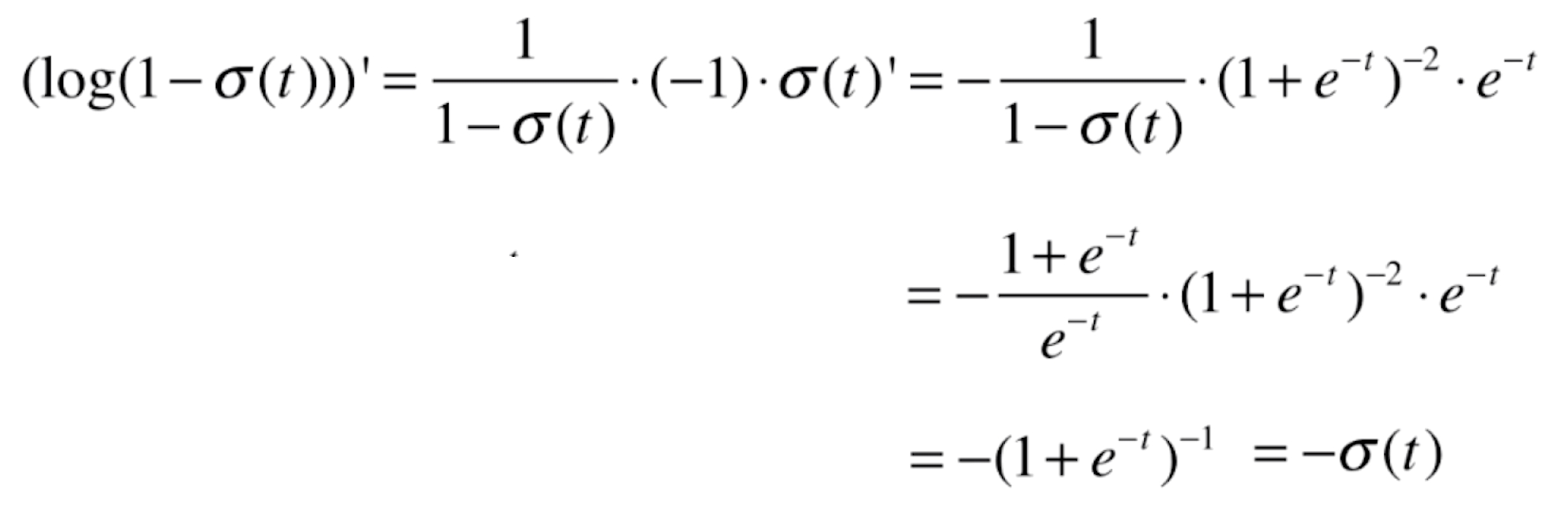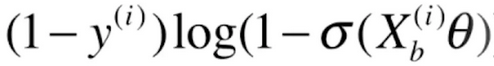的导数就为(这里同样也是复合函数求导，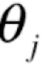跟随的特征就是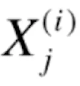)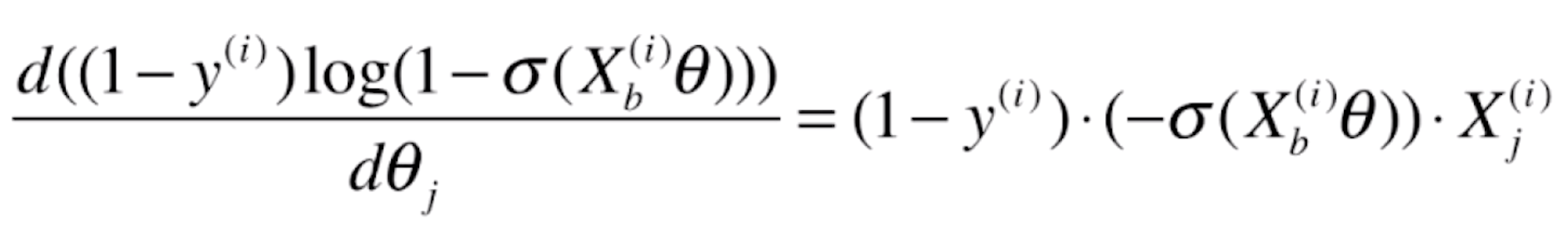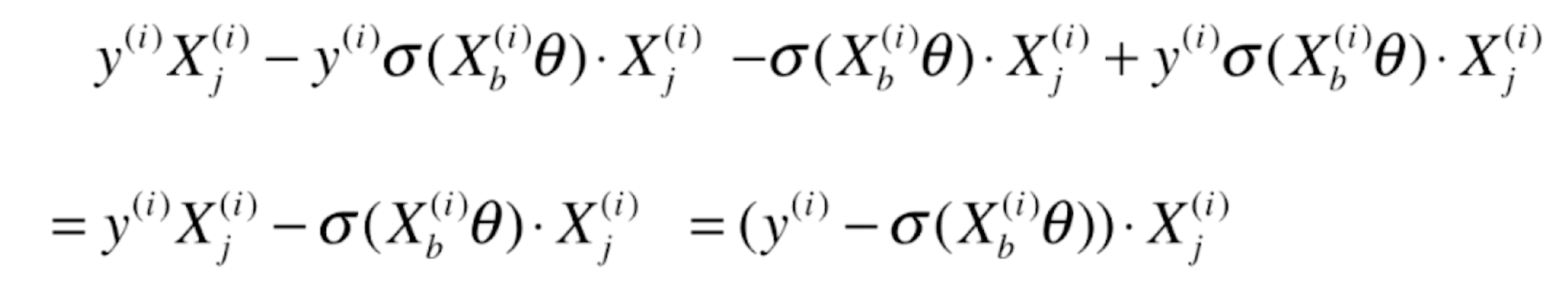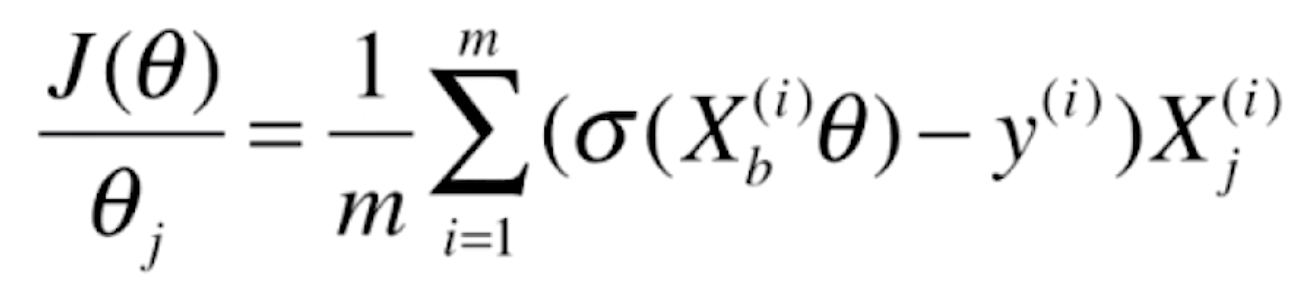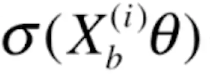就是我们逻辑回归的预测值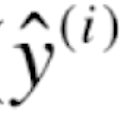，所以上式可以写为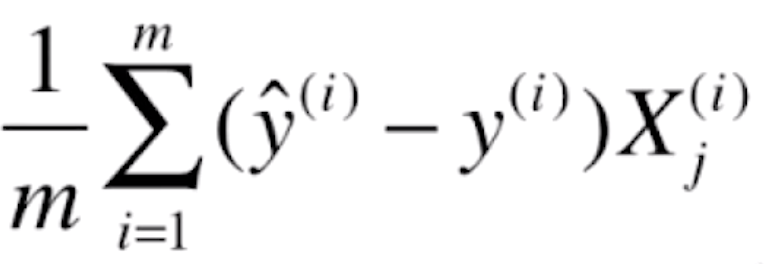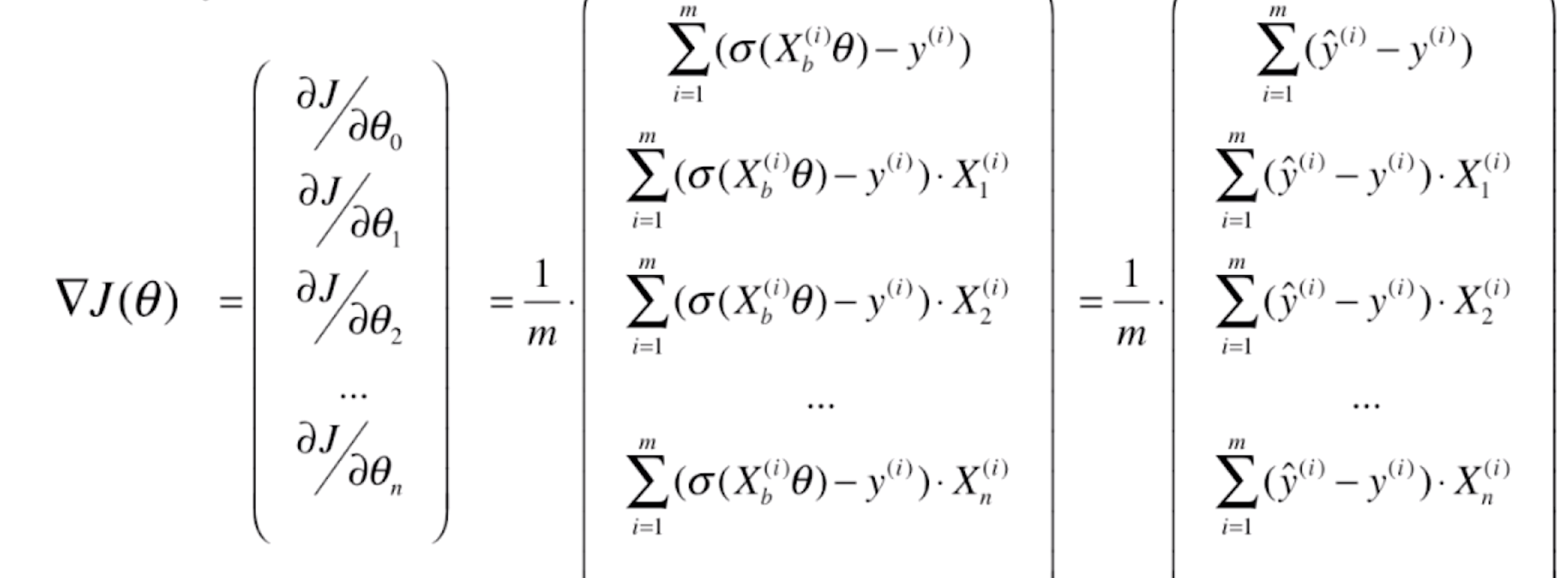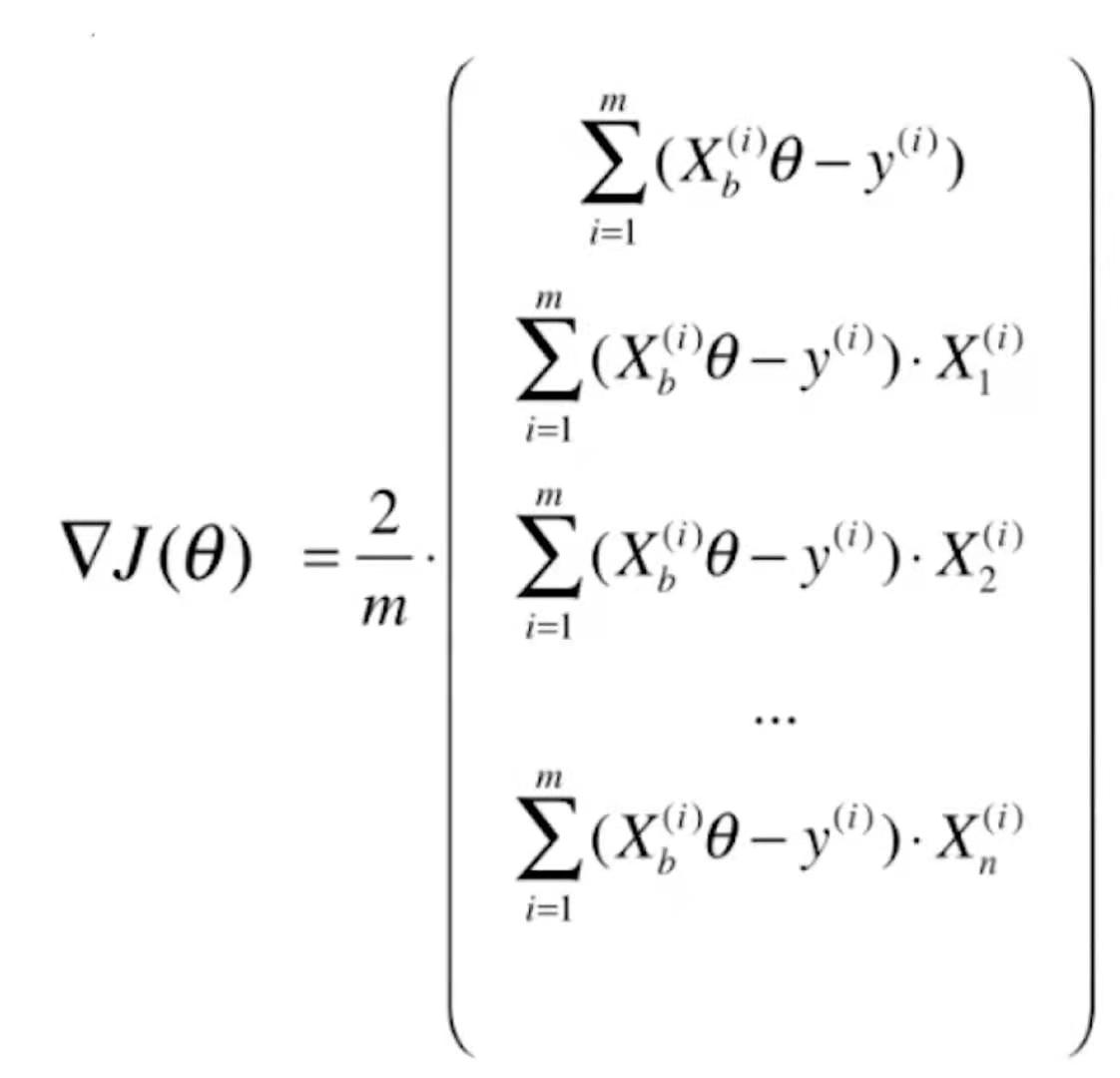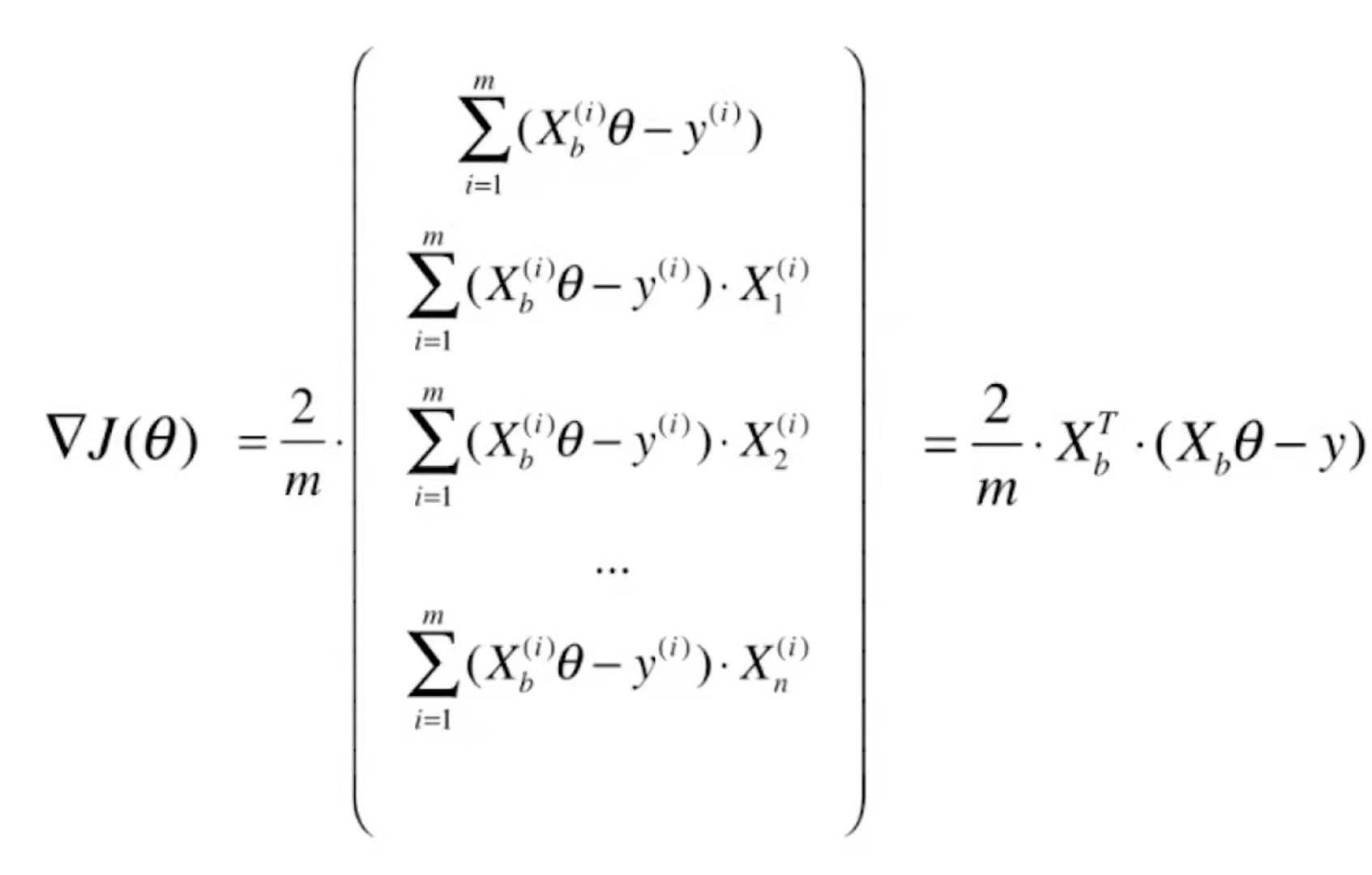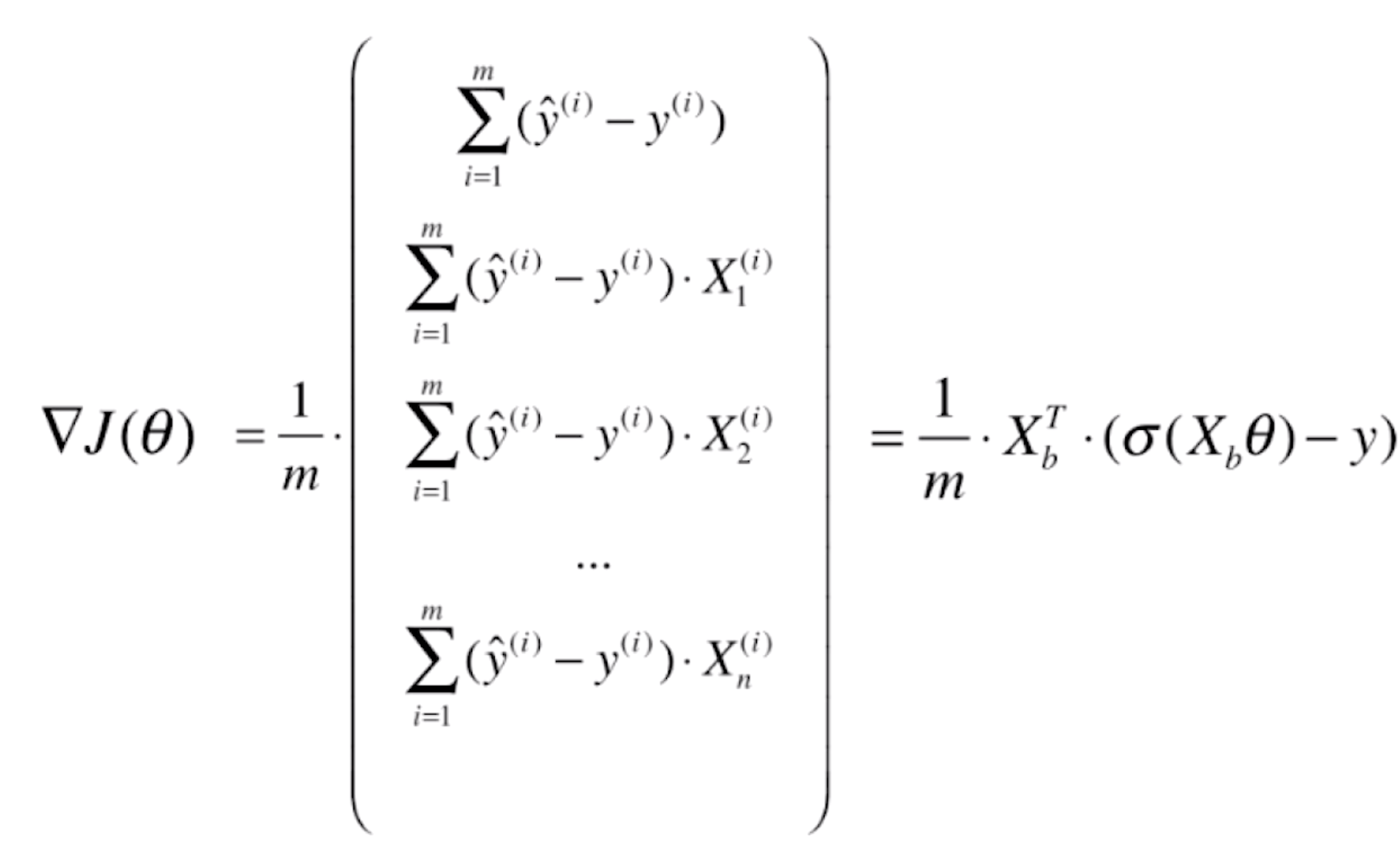```import numpy as np
from math import sqrt

def accuracy_score(y_true, y_predict):
"""计算y_true和y_predict之间的准确率"""
assert len(y_true) == len(y_predict),
"the size of y_true must be equal to the size of y_predict"

return np.sum(y_true == y_predict) / len(y_true)

def mean_squared_error(y_true, y_predict):
"""计算y_true和y_predict之间的MSE"""
assert len(y_true) == len(y_predict),
"the size of y_true must be equal to the size of y_predict"

return np.sum((y_true - y_predict)**2) / len(y_true)

def root_mean_squared_error(y_true, y_predict):
"""计算y_true和y_predict之间的RMSE"""

return sqrt(mean_squared_error(y_true, y_predict))

def mean_absolute_error(y_true, y_predict):
"""计算y_true和y_predict之间的MAE"""

return np.sum(np.absolute(y_true - y_predict)) / len(y_true)

def r2_score(y_true, y_predict):
"""计算y_true和y_predict之间的R Square"""
return 1 - mean_squared_error(y_true, y_predict) / np.var(y_true)```
```import numpy as np
from .metrics import accuracy_score

class LogisticRegression:

def __init__(self):
# 初始化LogisticRegression模型
self.coef = None  # 系数
self.interception = None  # 截距
self._theta = None

def _sigmoid(self, t):
return 1 / (1 + np.exp(-t))

def fit(self, X_train, y_train, eta=0.01, n_iters=1e4):
# 根据训练数据集X_train,y_train，使用梯度下降法训练Logistic Regression模型
assert X_train.shape == y_train.shape,
"X_train的列数必须等于y_train的长度"

def J(theta, X_b, y):
# 构建损失函数
p_hat = self._sigmoid(X_b.dot(theta))
try:
return - np.sum(y * np.log(p_hat) + (1 - y) * np.log(1 - p_hat)) / len(y)
except:
return float('inf')

def dJ(theta, X_b, y):
# 对theta求偏导数，获取梯度向量
return X_b.T.dot(self._sigmoid(X_b.dot(theta)) - y) / len(X_b)

def gradient_descent(X_b, y, initial_theta, eta, n_iters=1e4, epsilon=1e-8):
"""
梯度下降算法
:param X_b: 带虚拟特征1的自变量特征矩阵
:param y: 因变量向量
:param initial_theta: 初始的常数向量，这里需要注意的是真正待求的是常数向量，求偏导的也是常数向量
:param eta: 迭代步长、学习率
:param n_iters: 最大迭代次数
:param epsilon: 误差值
:return:
"""
theta = initial_theta
# 真实迭代次数
i_iter = 0
while i_iter < n_iters:
# 获取梯度
last_theta = theta
# 迭代更新theta，不断顺着梯度方向寻找新的theta
theta = theta - eta * gradient
# 计算前后两次迭代后的损失函数差值的绝对值
if abs(J(theta, X_b, y) - J(last_theta, X_b, y)) < epsilon:
break
# 更新迭代次数
i_iter += 1
return theta

X_b = np.hstack([np.ones((len(X_train), 1)), X_train])
initial_theta = np.zeros(X_b.shape)
self._theta = gradient_descent(X_b, y_train, initial_theta, eta, n_iters)
self.interception = self._theta
self.coef = self._theta[1:]
return self

def predict_proba(self, X_predict):
# 给定待预测数据集X_predict,返回表示X_predict的结果概率向量
assert self.interception is not None and self.coef is not None,
"开始预测前必须fit"
assert X_predict.shape == len(self.coef),
"预测的特征数必须与训练的特征数相等"
X_b = np.hstack([np.ones((len(X_predict), 1)), X_predict])
return self._sigmoid(X_b.dot(self._theta))

def predict(self, X_predict):
# 给定待预测数据集X_predict,返回表示X_predict的结果向量
assert self.interception is not None and self.coef is not None,
"开始预测前必须fit"
assert X_predict.shape == len(self.coef),
"预测的特征数必须与训练的特征数相等"
proba = self.predict_proba(X_predict)
return np.array(proba >= 0.5, dtype='int')

def score(self, X_test, y_test):
# 根据测试数据集X_test和y_test确定当前模型的准确度
y_predict = self.predict(X_test)
return accuracy_score(y_test, y_predict)

def __repr__(self):
return "LogisticRegression()"```

```import numpy as np
import matplotlib.pyplot as plt
from sklearn import datasets

if __name__ == "__main__":

X = iris.data
y = iris.target
# 鸢尾花数据集有3个分类，而逻辑回归只能进行二分类，所以我们去掉2这个特征
X = X[y < 2, :2]
y = y[y < 2]
print(X.shape)
print(y.shape)
plt.scatter(X[y == 0, 0], X[y == 0, 1], color='red')
plt.scatter(X[y == 1, 0], X[y == 1, 1], color='blue')
plt.show()```

``````(100, 2)
(100,)``````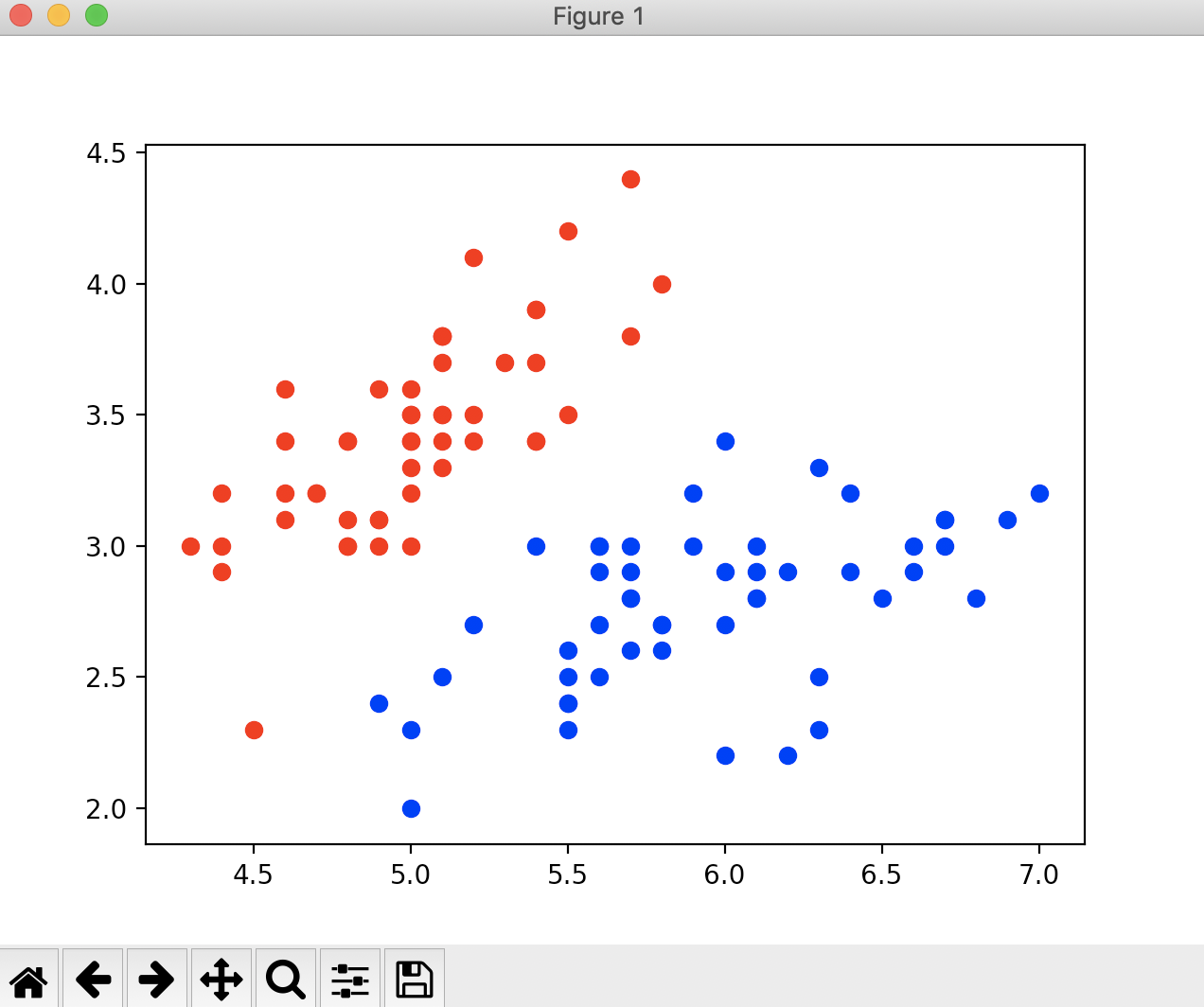```import numpy as np
import matplotlib.pyplot as plt
from sklearn import datasets
from playLA.model_selection import train_test_split
from playLA.LogisticRegression import LogisticRegression

if __name__ == "__main__":

X = iris.data
y = iris.target
# 鸢尾花数据集有3个分类，而逻辑回归只能进行二分类，所以我们去掉2这个特征
X = X[y < 2, :2]
y = y[y < 2]
print(X.shape)
print(y.shape)
# plt.scatter(X[y == 0, 0], X[y == 1, 1], color='red')
# plt.scatter(X[y == 1, 0], X[y == 1, 1], color='blue')
# plt.show()

X_train, X_test, y_train, y_test = train_test_split(X, y, seed=666)
log_reg = LogisticRegression()
log_reg.fit(X_train, y_train)
# 查看分类准确度
print(log_reg.score(X_test, y_test))
# 查看测试数据集每一个元素的概率值
print(log_reg.predict_proba(X_test))
# 查看分类结果
print(y_test)
# 查看预测结果
print(log_reg.predict(X_test))```

``````(100, 2)
(100,)
1.0
[0.92972035 0.98664939 0.14852024 0.01685947 0.0369836  0.0186637
0.04936918 0.99669244 0.97993941 0.74524655 0.04473194 0.00339285
0.26131273 0.0369836  0.84192923 0.79892262 0.82890209 0.32358166
0.06535323 0.20735334]
[1 1 0 0 0 0 0 1 1 1 0 0 0 0 1 1 1 0 0 0]
[1 1 0 0 0 0 0 1 1 1 0 0 0 0 1 1 1 0 0 0]``````

```import numpy as np
import matplotlib.pyplot as plt
from sklearn import datasets
from playLA.model_selection import train_test_split
from playLA.LogisticRegression import LogisticRegression

if __name__ == "__main__":

X = iris.data
y = iris.target
# 鸢尾花数据集有3个分类，而逻辑回归只能进行二分类，所以我们去掉2这个特征
X = X[y < 2, :2]
y = y[y < 2]
print(X.shape)
print(y.shape)
# plt.scatter(X[y == 0, 0], X[y == 0, 1], color='red')
# plt.scatter(X[y == 1, 0], X[y == 1, 1], color='blue')
# plt.show()

X_train, X_test, y_train, y_test = train_test_split(X, y, seed=666)
log_reg = LogisticRegression()
log_reg.fit(X_train, y_train)
# 查看分类准确度
print(log_reg.score(X_test, y_test))
# 查看测试数据集每一个元素的概率值
print(log_reg.predict_proba(X_test))
# 查看分类结果
print(y_test)
# 查看预测结果
print(log_reg.predict(X_test))
# 查看逻辑回归的系数
print(log_reg.coef)
# 查看逻辑回归的截距
print(log_reg.interception)```

``````(100, 2)
(100,)
1.0
[0.92972035 0.98664939 0.14852024 0.01685947 0.0369836  0.0186637
0.04936918 0.99669244 0.97993941 0.74524655 0.04473194 0.00339285
0.26131273 0.0369836  0.84192923 0.79892262 0.82890209 0.32358166
0.06535323 0.20735334]
[1 1 0 0 0 0 0 1 1 1 0 0 0 0 1 1 1 0 0 0]
[1 1 0 0 0 0 0 1 1 1 0 0 0 0 1 1 1 0 0 0]
[ 3.01796521 -5.04447145]
-0.6937719272911228``````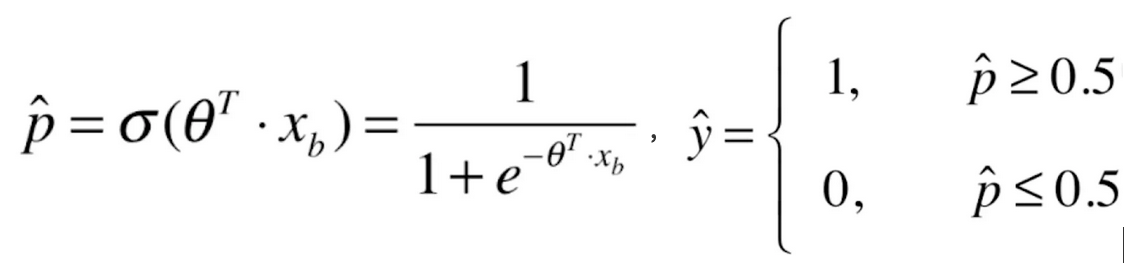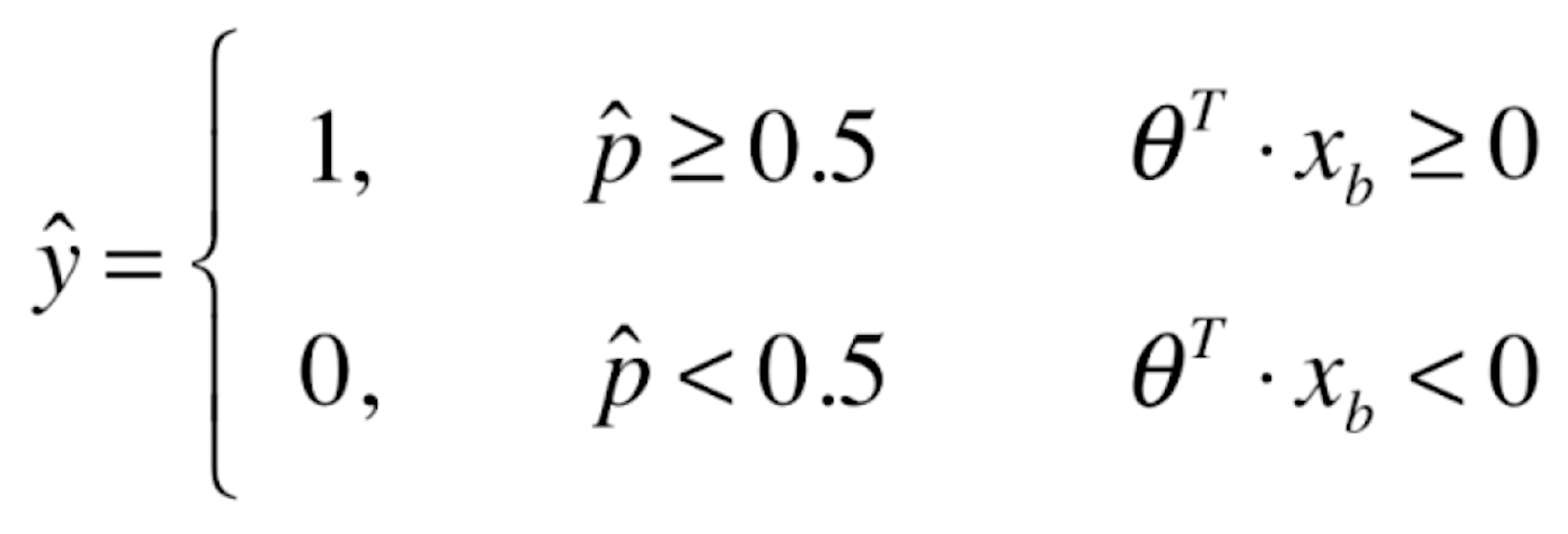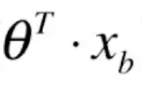≥0的时候，我们的概率估计值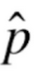≥0.5，此时我们将新来的样本x分类为y=1；当<0的时候，我们的概率估计值<0.5，此时我们将新来的样本x分类为y=0。换句话说，我们为新来的样本分类为1还是0，这个边界点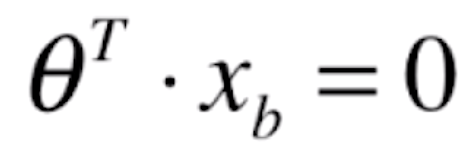，这个位置就被称为决策边界两个向量进行点乘，它同时代表的是一条直线。如果X有两个特征，那么就可以写成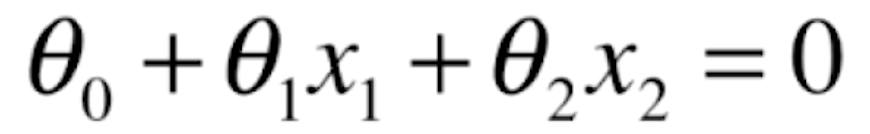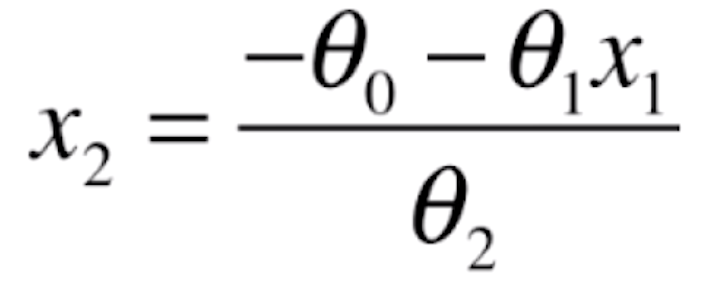```def x2(x1):
return (- log_reg.coef * x1 - log_reg.interception) / log_reg.coef

x1_plot = np.linspace(4, 8, 1000)
x2_plot = x2(x1_plot)
plt.scatter(X[y == 0, 0], X[y == 0, 1], color='red')
plt.scatter(X[y == 1, 0], X[y == 1, 1], color='blue')
plt.plot(x1_plot, x2_plot)
plt.show()```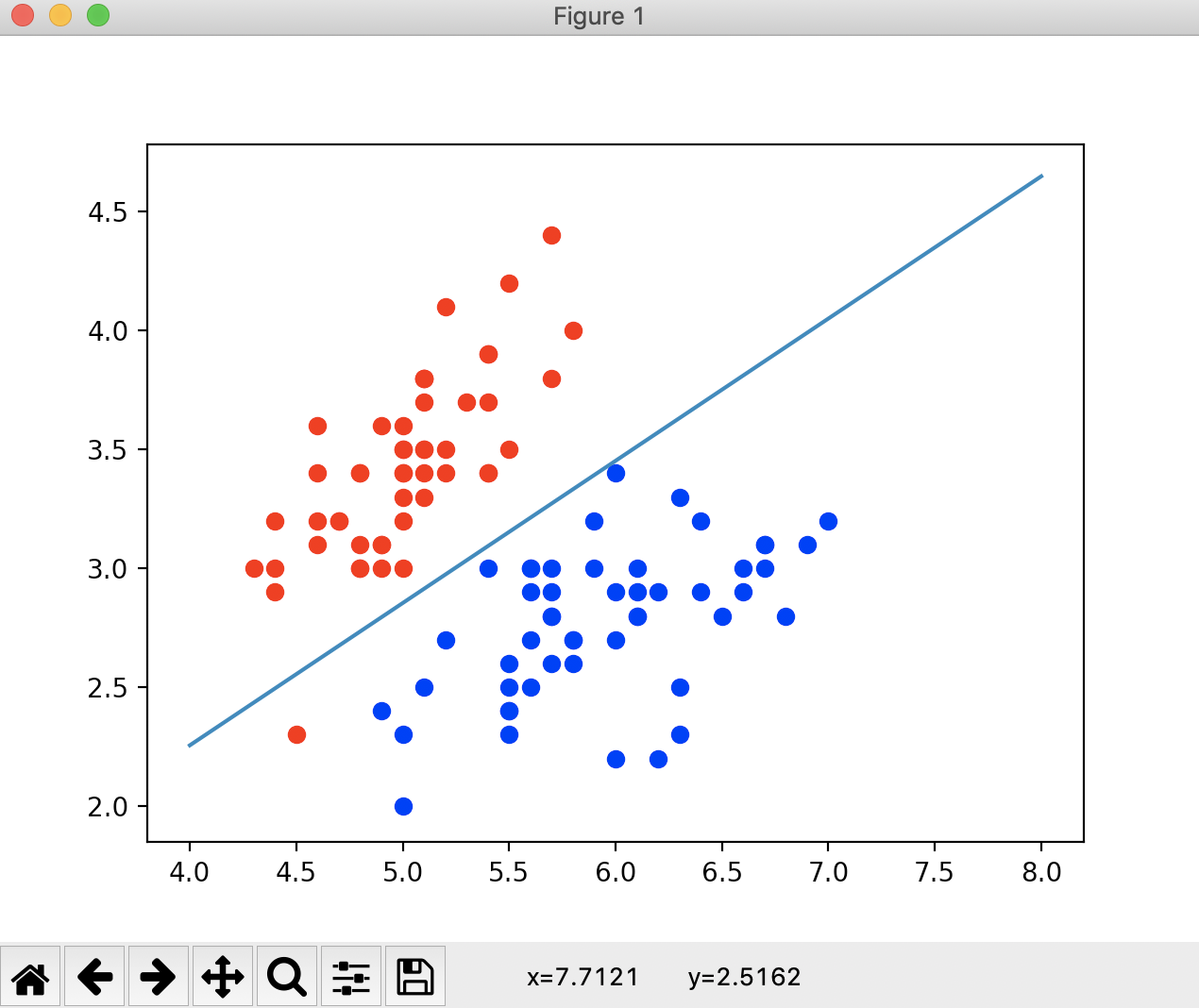```def x2(x1):
return (- log_reg.coef * x1 - log_reg.interception) / log_reg.coef

x1_plot = np.linspace(4, 8, 1000)
x2_plot = x2(x1_plot)
# plt.scatter(X[y == 0, 0], X[y == 0, 1], color='red')
# plt.scatter(X[y == 1, 0], X[y == 1, 1], color='blue')
# plt.plot(x1_plot, x2_plot)
# plt.show()

plt.scatter(X_test[y_test == 0, 0], X_test[y_test == 0, 1], color='red')
plt.scatter(X_test[y_test == 1, 0], X_test[y_test == 1, 1], color='blue')
plt.plot(x1_plot, x2_plot)
plt.show()```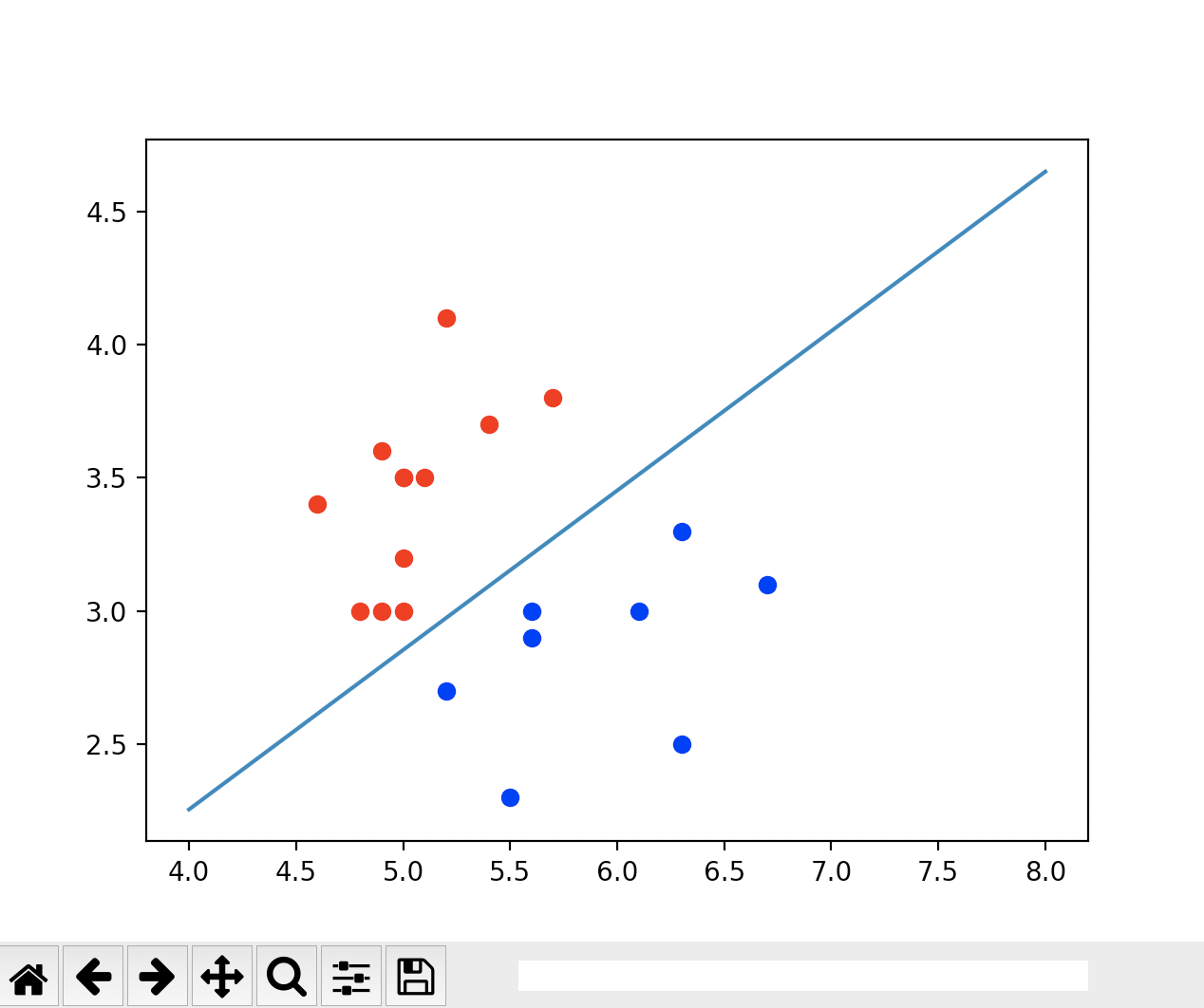### 吐槽小黑屋()

* 这里是“吐槽小黑屋”，所有人可看，只保留当天信息。

• ###Erlo.vip2021-09-28 04:11:38Hello、欢迎使用吐槽小黑屋，这就是个吐槽的地方。
• 返回顶部### 给这篇文章打个标签吧~

棒极了 糟糕透顶 好文章 PHP JAVA JS 小程序 Python SEO MySql 确认